# Decimals

Here you will learn about decimal numbers including how to read and write them, understand their place value, and strategies to compare them.

Students will first learn about decimals in 4th grade math as part of their work in number and operations with fractions and extend their knowledge as they work through number systems in middle school.

## What are decimals?

Decimals are numbers that have a whole part and a fractional part that are separated by a decimal point. Our decimal system splits whole numbers into tenths, hundredths, thousandths, and so on.

This decimal place value chart can help you understand decimal place value.

Step-by-step guide: Decimal place value

Step-by-step guide: Decimal places

You can convert fractions to decimals and decimals to fractions.

Fractions as decimals \hspace{1.7cm}

Fraction → \cfrac{3}{10}

Decimal → 0.3

The 3 is placed in the tenths
column. Since there are no
digits in the hundredths and
thousandths place it can be
left blank or 0’ s can be

Fraction → \cfrac{3}{100}

Decimal → 0.03

The 3 is placed in the
hundredths column and a 0
in the tenths column. Since
there are no digits in the
thousandths place it can
be left blank or a 0 can be

Fraction → \cfrac{3}{1000}

Decimal → 0.003

The 3 is placed in the
thousandths column and
0’ s are placed in both the
tenths and the
hundredths columns.

Decimals as fractions \hspace{1.7cm}

Decimal → 0.43

43 is the numerator of the fraction and 100
is the denominator because the last digit of
the decimal is in the hundredths column of
the place value chart,
0.43 =\cfrac{43}{100}

Decimal → 0.009

9 is the numerator of the fraction and
1000 is the denominator because the 9 is
in the thousandths column of the place
value chart,
0.009=\cfrac{9}{1000}

You can compare decimal numbers using a place value chart or converting them to fractions.

Comparing decimals with equivalent fractions

Let’s compare 0.009, \, 0.09 and 0.9 by listing them in order from least to greatest.

0.009 is 9 thousandths → \cfrac{9}{1000}

0.09 is 9 hundredths → \cfrac{9}{100}

0.9 is 9 tenths → \cfrac{9}{10}

First, use a common denominator that is a power of ten.

The common denominator of \cfrac{9}{100} \, , \, \cfrac{9}{1000} \, , \, \cfrac{9}{10} \, is 1000.

\cfrac{9}{100} \, =\cfrac{9\times 10}{100\times 10} \, =\cfrac{90}{1000}

\cfrac{9}{1000} \, already has a denominator of 1000

\cfrac{9}{10} \, =\cfrac{9\times 100}{10\times 100} \, =\cfrac{900}{1000}

The fractions are now easy to compare.

From least to greatest the fractions are:

\cfrac{9}{1000} \, , \, \cfrac{90}{1000} \, , \, \cfrac{900}{1000}

So, the decimal numbers in order from least to greatest are:

0.9, 0.09, 0.009

0.009 < 0.09 < 0.9

Step by step guide: Comparing decimals

You can place decimals on a number line to help visualize the value of decimals.

Decimal on a number line

A decimal number line is a number line that includes decimal increments and can help
you to visualize decimal numbers.

Here is an example of a decimal number line that is in increments of tenths from 0 to 1.

If you zoom in on the number line between 0.4 and 0.5 that goes up in steps of 0.01
you can position the decimal 0.472 more accurately.

The position has been estimated between 0.47 and 0.48, but closer to 0.47.

Step by step guide: Decimal number line

You can perform calculations on decimal numbers like you would with multi-digit numbers.

Add: 3.87 + 4.21 = \, ?

● Line up the decimal points
● Add 0' s as placeholders where necessary
digit numbers

Subtract: 5.6- 3.79 = \, ?

● Line up the decimal points
● Add 0' s as placeholders where necessary
● Subtract in the same way as subtracting
multi-digit numbers

Step by step guide: Adding and subtracting decimals

Multiplying and dividing decimals \hspace{1.7cm}

Multiply: 0.34\times 3 = \, ?

● Put the number with the most amount of
digits on top
● Multiply in the same way as multiplying
multi-digit numbers.
● Count up the total digits to the right of
the decimal point

Divide: 1.10\div 2 = \, ?

● Line up the decimal point of the dividend
with the decimal point of the quotient
● Divide using long division

Step by step guide: Multiplying and dividing decimals

### What are decimals?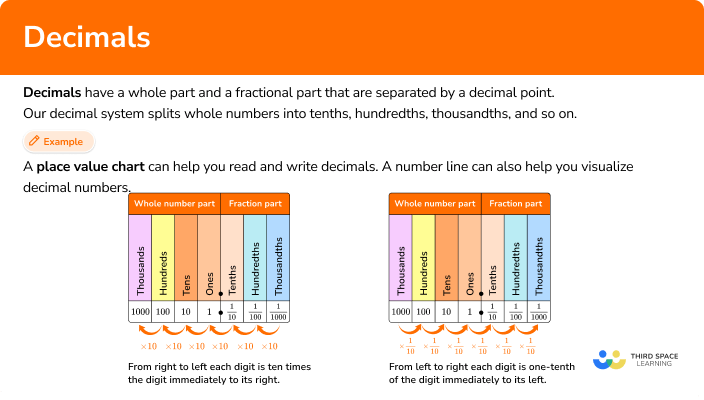## Common Core State Standards

• Grade 4 – Number and Operations Fractions (4.NF.C.7)
Compare two decimals to hundredths by reasoning about their size. Recognize that comparisons are valid only when the two decimals refer to the same whole. Record the results of comparisons with the symbols >, =, or < , and justify the conclusions, example, by using a visual model.

• Grade 5 – Number and Operations Base Ten (5.NBT.A.3)
Read, write, and compare decimals to thousandths.

• Grade 5 – Number and Operations in Base Ten (5.NBT.A.1)
Recognize that in a multi-digit number, a digit in one place represents 10 times as much as it represents in the place to its right and \frac{1}{10} of what it represents in the place to its left.

• Grade 5 – Number and Operations in Base Ten (5.NBT.B)
Perform operations with multi-digit whole numbers and with decimals to hundredths.

• Grade 6: The Number System (6.NS.B.3)
Fluently add, subtract, multiply, and divide multi-digit decimals using the standard algorithm for each operation.

## How to write decimal numbers

In order to write a fraction as a decimal:

1. Write the fraction in words.
2. Place the numerator of the fraction on the place value chart.
3. Write the decimal number.

In order to write a decimal as a fraction:

1. Write the decimal in words.
2. The digits of the decimal is the numerator, and the denominator is the column of the last digit on the place value chart.
3. Write the fraction and simplify if possible.

## Decimals examples

### Example 1: write the fraction as a decimal

Change \cfrac{47}{100} \, to a decimal number.

1. Write the fraction in words.

\cfrac{47}{100} \, is 47 hundredths.

2Place the numerator of the fraction in the correct spaces on the place value chart.

3Write the decimal number.

\cfrac{47}{100} \, as a decimal is 0.47.

### Example 2: writing a decimal as a fraction

Write 0.017 as a fraction.

Write the decimal in words.

The digits of the decimal is the numerator, and the denominator is the column of the last digit on the place value chart.

Write the fraction.

### Example 3: comparing decimals using a place value chart

Use the place value chart to list these decimal numbers in order from least to greatest.

0.05, 0.02, 0.205, 0.052, 0.502

Put the number on the place value chart.

Add zeros as place holders as necessary.

Next, move on to the hundredths place and then the thousandths place.

Write the decimal numbers in the correct order.

### Example 4: comparing decimals using equivalent fractions

List the decimals in order from least to greatest, using equivalent fractions.

0.4, \, 0.004, \, 0.44, \, 0.04

Rewrite the decimals as fractions

Use equivalent fractions to get a common denominator.

Write the fraction numbers in order or use \textbf{>, <, =} to compare them.

Write the decimal numbers in order or use \textbf{>, <, =} to compare them.

### Example 5: add decimal numbers

5.4 + 1.27

Stack the numbers and line up the decimal points (and place value).

Add each column from right to left and regroup the numbers when necessary.

### Example 6: multiply decimal numbers

Multiply the decimal numbers,

3.2\times 4.03

Stack the number with the most digits on top.

Multiply as if the numbers were multi-digit whole numbers, regrouping when necessary.

Count the number of digits after the decimal point for each factor.

Put the same number of digits after the decimal point for the product.

### Teaching tips for decimals

• Using visual models such as number lines or place value representation helps students build number sense as they learn decimal place values.

• Although decimal worksheets have their place in the classroom, utilizing online platforms for game playing helps to motivate students and also provides time for them to practice.

• Reinforcing placeholder 0 ’s makes the decimal numbers have the same digits so that students can easily compare them. It also builds understanding for decimal operations.

### Easy mistakes to make

• Confusing decimal numbers
For example,
When comparing 0.50 and 0.05 and thinking they are both 5 hundredths or 5 tenths.

• Forgetting to use to placeholder \bf{0} ’s when comparing decimals

• Adding \bf{0} ’s placeholders in the wrong place
For example,
Adding the 0 ’s as placeholders before the 9 in the decimal number 0.9 instead of after the 9.

### Practice decimals questions

1. Which decimal represents the fraction \cfrac{17}{100}?

0.170.0170.00170.07\cfrac{17}{100} \, is 17 hundredths.

Place 1 in the tenths column and 7 in the hundredths column.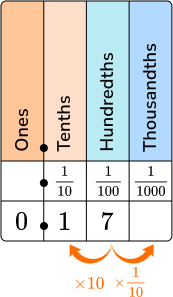The decimal number is 0.17.

2. Which fraction represents the decimal 0.092?

\cfrac{92}{10}\cfrac{92}{100}\cfrac{92}{1000}\cfrac{92}{1000}0.092 is ninety-two thousandths. Place the decimal number on the place value chart, 0 in the tenths column, 9 in the hundredths column and 2 in the thousandths column.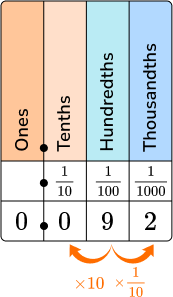92 is the numerator of the fraction, and 1000 will be the denominator of the fraction.

\cfrac{92}{1000}

3. Write the decimals in order from least to greatest using a place value chart.

0.46, \, 0.06, \, 0.5, \, 0.4

0.4, \, 0.46, \, 0.5, \, 0.060.06, \, 0.5, \, 0.46, \, 0.40.06, \, 0.4, \, 0.46, \, 0.50.5, \, 0.46, \, 0.4, \, 0.06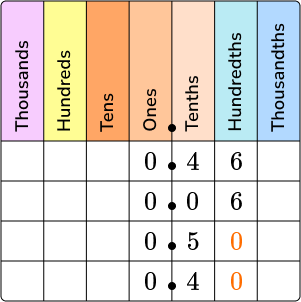You can see from the chart that 0.06 has the smallest value and 0.50 has the greatest value.

You can also see from the hundredths column that 0.46 is larger than 0.40.

The numbers in order from least to greatest are:

0.06, \, 0.40, \, 0.46, \, 0.50

0.06 < 0.40 < 0.46 < 0.50

4. Write the decimals in order from least to greatest using equivalent fractions.

0.66, \, 0.116, \, 0.16, \, 0.6

\cfrac{66}{100}, \, \cfrac{116}{100}, \, \cfrac{16}{100}, \, \cfrac{6}{100} \; (0.66, \, 0.116, \, 0.16, \, 0.6)\cfrac{6}{100}, \, \cfrac{66}{100}, \, \cfrac{116}{1000}, \, \cfrac{16}{100} \; (0.6, \, 0.66, \, 0.116, \, 0.16)\cfrac{60}{1000}, \, \cfrac{116}{1000}, \, \cfrac{160}{1000}, \, \cfrac{660}{1000} \; (0.6, \, 0.116, \, 0.16, \, 0.66)\cfrac{116}{1000}, \, \cfrac{160}{1000}, \, \cfrac{600}{1000}, \, \cfrac{660}{1000} \; (0.116, \, 0.16, \, 0.6, \, 0.66)Write each decimal as a fraction.

0.66 is 66 hundredths which is \, \cfrac{66}{100}.

0.116 is 116 thousandths which is \, \cfrac{116}{1000}.

0.16 is 16 hundredths which is \, \cfrac{16}{100}.

0.6 is 6 tenths which is \cfrac{6}{10}.

The common denominator is 1000; use equivalent fractions to rewrite fractions with the denominator 1000.

\cfrac{66}{100}=\cfrac{66\times 10}{100\times 10}=\cfrac{660}{1000}

\cfrac{116}{1000} \, has a denominator of 1000.

\cfrac{16}{100}=\cfrac{16\times 10}{100\times 10}=\cfrac{160}{1000}

\cfrac{6}{10}=\cfrac{6\times 100}{10\times 100}=\cfrac{600}{1000}

The fractions are easier to compare when they have the same denominator.

The fractions in order from least to greatest are:

\cfrac{116}{1000}, \, \cfrac{160}{1000}, \, \cfrac{600}{1000}, \, \cfrac{660}{1000}

Ordering decimals in order from least to greatest are:

0.116, \, 0.16, \, 0.6, \, 0.66

6.4710.0710.76.11Line up the decimal points.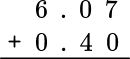Add placeholder 0 ’s where necessary. Then add the numbers the same way you would add multi-digit numbers.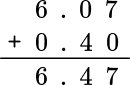6. Multiply: 7.3\times 0.4.

28.1229.22.920.292Place the number with the greatest number of digits on top. Multiply the numbers the way you would multi-digit numbers.

Count the total number of digits to the right of the decimal point in both numbers. Make sure the answer has the same number of total digits to the right of the decimal point.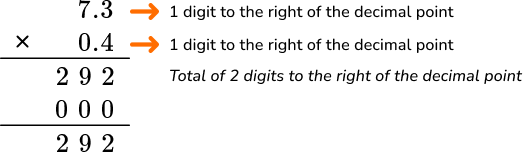Move the decimal point two places so that there is 2 digits to the right of the decimal point.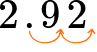## Decimals FAQs

Are there other strategies for comparing decimals numbers?

Yes, using a number line is another visual strategy to compare decimals. Comparing 0.2 and 0.6 on the number line, you can see that 0.2 is closer to 0 and 0.6 is further to the right. This means that 0.6 is the larger number. The further right a number is on the number line the larger it is.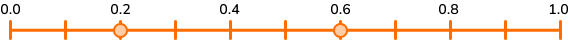Why is place value of decimals important?

Decimal place value is important because it provides a foundation for the decimal system and decimal operations.

How are decimals and fractions similar?

Decimals and fractions are very similar because they both have a whole part and a fraction part.

What are decimal fractions?

A decimal fraction is when the denominator of the fraction is a power of 10.
For example, \cfrac{3}{100} \, is a decimal fraction because 100 is 10^2.

Are decimal numbers similar to mixed numbers?

Yes, decimal numbers bigger than 1 are similar to mixed numbers.
For example, 1.3 can be represented as the mixed number 1\cfrac{3}{10}.

The digits to the left of the decimal point represent ones, tens, hundreds, etc. The numbers to the right of the decimal point represent tenths, hundredths, thousandths, etc.

## Still stuck?

At Third Space Learning, we specialize in helping teachers and school leaders to provide personalized math support for more of their students through high-quality, online one-on-one math tutoring delivered by subject experts.

Each week, our tutors support thousands of students who are at risk of not meeting their grade-level expectations, and help accelerate their progress and boost their confidence.